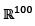# Let W1 and W2 be subspaces of the real vector spacedefined byW1 = {(x1,x2, ...,x100) : xi = 0 if i is divisible by 4},W2 = { (x1;x2, ....x100) : xi = 0 if i is divisible by 5}.Then the dimension of W1 ∩ W2 is ____Correct answer is between '60,60'. Can you explain this answer? Related Test: Math - 2019 Past Year Paper

## Can you answer this question?

People are searching for an answer to this question.